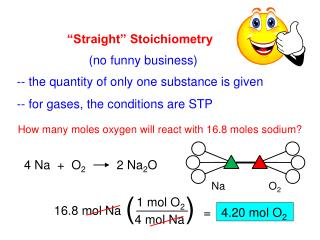# 4 Na + O 2 2 Na 2 O - PowerPoint PPT PresentationDownload Presentation4 Na + O 2 2 Na 2 O

Download Presentation## 4 Na + O 2 2 Na 2 O

- - - - - - - - - - - - - - - - - - - - - - - - - - - E N D - - - - - - - - - - - - - - - - - - - - - - - - - - -
##### Presentation Transcript

1. 4 Na + O22 Na2O ( ) “Straight” Stoichiometry (no funny business) -- the quantity of only one substance is given -- for gases, the conditions are STP How many moles oxygen will react with 16.8 moles sodium? Na O2 1 mol O2 16.8 molNa = 4.20 mol O2 4 molNa

2. ( ) ( ) ( ) ( ) 2 atoms O 1 m’c O2 At STP, how many molecules of oxygen react with 632 dm3 butane (C4H10)? C4H10 O2 1 4 5 2 13 8 10 __ CO2 + __ H2O __ C4H10 + __ O2 1 mol C4H10 13 mol O2 632 dm3 C4H10 6.02 x 1023 m’c O2 22.4 dm3 C4H10 1 mol O2 2 mol C4H10 = 1.10 x 1026 m’c O2 Suppose the question had been “how many ATOMS of O…” 1.10 x 1026 m’c O2 = 2.20 x 1026 at. O

3. ( ) ( ) ( ) ( ) 2 atoms O 1 m’c O2 At STP, how many molecules of oxygen react with 632 dm3 butane (C4H10)? C4H10 O2 13 2 8 10 __ CO2 + __ H2O __ C4H10 + __ O2 1 mol C4H10 13 mol O2 632 dm3 C4H10 6.02 x 1023 m’c O2 22.4 dm3 C4H10 1 mol O2 2 mol C4H10 = 1.10 x 1026 m’c O2 Suppose the question had been “how many ATOMS of O…” 1.10 x 1026 m’c O2 = 2.20 x 1026 at. O

4. ( ) ( ) ( ) How many grams potassium will react with 465 grams nickel(II) phosphide? Ni3P2 K 6 3 Ni + K3P 2 1 mol Ni3P2 6 mol K 465 g Ni3P2 39.1 g K 1 mol K 1 mol Ni3P2 238.1 g Ni3P2 = 458 g K K + Ni3P2

5. Limiting Reactants (a.k.a., Limiting Reagents) limiting reactant (LR): the reactant that runs out first -- Amount of EVERYTHING depends on the LR. Any reactant you don’t run out of is an excess reactant (ER).

6. For the generic reaction RA + RBP, assume that the amounts of RA and RB are given. Should you use RA or RB in your calculations? How to Find the Limiting Reactant 1. Calc. # of mol of RA and RB you have. 2. Divide by the respective coefficients in balanced equation. 3. Reactant having the smaller result is the LR.

7. 2 H2(g) + O2(g) 2 H2O(g) ( ) . . _ _ . . ( ( ) ) ( ) How many g H2O are formed? 13 g H2 80. g O2 1 mol H2 = 3.25 13 g H2 = 6.5 mol H2 (HAVE) 2 2 g H2 LR = O2 1 mol O2 80 g O2 1 = 2.50 = 2.5 mol O2 (HAVE) 32 g O2 “Oh, bee- HAVE !” (And start every calc. with the LR.) O2 H2O 2 mol H2O 18 g H2O 2.5 mol O2 = 90. g H2O 1 mol H2O 1 mol O2

8. 2 Fe(s) + 3Cl2(g) 2 FeCl3(s) ( ) . . _ _ . . ( ) ( ( ) ) 223 g Fe 179 L Cl2 At STP, what is the limiting reactant? LR 1 mol Fe = 2.0 223 g Fe = 4.0 mol Fe (HAVE) 2 55.8 g Fe = Fe 1 mol Cl2 179 L Cl2 3 = 2.66 = 8.0 mol Cl2 (HAVE) 22.4 L Cl2 What mass of FeCl3 is produced? Fe FeCl3 (“Oh, bee-HAVE!”) 2 mol FeCl3 162.3 g FeCl3 = 649 g FeCl3 4.0 mol Fe 1 mol FeCl3 2 mol Fe